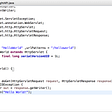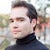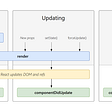# CoDEVIANT #14 (9/30/20) — The 2020 Terrorscape

• N is an integer within the range [1..2,147,483,647].
`function solution(N) {    let array = N.toString(2).split("")    let counter = 0     let max = 0    let spareArray = []    array.forEach(i => {        if(Number(i) === 1) {            spareArray.push(max)            counter = 0        } else {            counter++            max = counter        }    })        return spareArray.sort()[spareArray.length - 1]}`
• Turn the number into a binary:
I accomplish this by using toString(2) on the N argument passed into the function.
• I then turn the binary number into an array
I accomplish this by chaining the split(‘’) method onto binary derived from the step above
• And we package that guy into the variable named array
• I set counter and max to equal 0
• I create an empty array called spareArray.
`export function solution(N) {      const binaryString = N.toString(2);      const start = '10';  const end = '01';      let startIndex = binaryString.indexOf(start);      let endIndex = -1;      let binaryGap = 0;    // Remember that N is the    // input number, and when you iterate over its binary representation, its always    // log(N).      while (startIndex >= 0) {          endIndex = binaryString.indexOf(end, startIndex);          if (endIndex < 0) {              break;          }          const tempGap = endIndex - startIndex;          binaryGap = tempGap > binaryGap ? tempGap : binaryGap;      startIndex = binaryString.indexOf(start, endIndex + 1);             endIndex = -1;      }  return binaryGap;}`
• start is ‘10’
• end is ‘01’
• binaryString is like how we handled N in our solution.
• We have startIndex equal the indexOf where ‘10’ (aka start) is at in the binaryString.
`if(endIndex < 0 ) {    break;}`
• N and K are integers within the range [0..100];
• each element of array A is an integer within the range [−1,000..1,000].
`function solution(A, K) {    let arr = []    if(K === A.length) {        arr = A    } else {        A.forEach((i, index) => {            let numba = index - K            if(numba < 0) {                numba = index + (K - 1)            }            arr.push(A[numba])        })    }    return arr}`
• We make an empty array called arr.
• If K equals the length of the A array, arr will be the A array.
• If K isn’t the same as A’s length, then for each element in A, we’ll have numba equal the index of the A-element being looped through at that moment minus K.
• If numba is less than 0, numba is going to equal { index + (K — 1) }
• Then we push A[numba] into the arr array.
• Then we return the arr array.
`function solution(A, K) {         K = (A.length > K) ? K : K % A.length;         var d = A.slice(0, A.length - K);        var e = A.splice(A.length - K);        return e.concat(d); }`
• If the length of A is more than the argument passed in for K at the outset, then K stays as it is. Otherwise, K is going to be the remainder of K divided by A’s length.
• d equals a slice of the A-array starting at the zero-index position ending at the index before the A.length-K .
• e equals a splice of the A-array passing in A.length — K.
This means that we will get the values of A from the zero-index position of the argument forward.
• We return e concatenated with d.

--

--

--

is a web developer, opera singer, actor, and lover of cats. (adrian-rosales.tech)

Love podcasts or audiobooks? Learn on the go with our new app.

## How to Use React + Webpack for Front End Development in Salesforce Lightning## Being better at updating npm dependencies — part 1 of 2## Creating a WebSocket in Java…..## How to Write a smart contract## Sitemap with Next.js## How to fulfill all your promises — Promise.all## Eclipse IDE Server 使用筆記-02## Fragmentation (#3)is a web developer, opera singer, actor, and lover of cats. (adrian-rosales.tech)

## Data types in TypeScript. The Complete TypeScript Developer Course 2022 s.## React Component’s Lifecycle